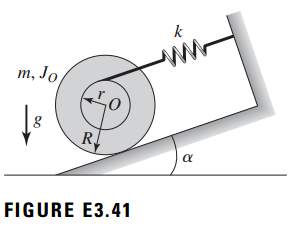### Create an Account

Home / Questions / The uniform concentric cylinder of radius R rolls without slipping on the inclined surface...

# The uniform concentric cylinder of radius R rolls without slipping on the inclined surface as shown in Figure E341 The cylinder has another cylinder of radius r concentrically attached

The uniform concentric cylinder of radius R rolls without slipping on the inclined surface as shown in Figure E3.41. The cylinder has another cylinder of radius r < r="" concentrically="" attached="" to="" it.="" the="" smaller="" cylinder="" has="" a="" cable="" wrapped="" around="" it.="" the="" other="" end="" of="" the="" cable="" is="" fixed.="" the="" cable="" is="" parallel="" to="" the="" inclined="" surface.="" if="" the="" stiffness="" of="" the="" cable="" is="" k,="" the="" mass="" and="" rotary="" inertia="" of="" the="" two="" attached="" cylinders="" are="" m="" and="" jo,="" respectively,="" then="" determine="" an="" expression="" for="" the="" natural="" frequency="" of="" the="" system="" in="" hz.="" the="" length="" of="" the="" unstretched="" spring="" is="">Jun 25 2020 View more View LessSubscribe To Get Solution